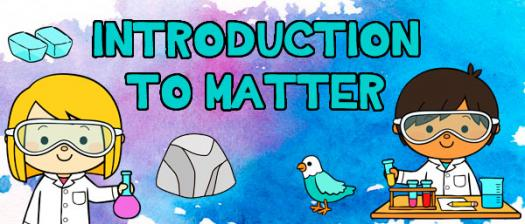# Introduction To Matter

13 QuestionsSettings.

• 1.
Wood is matter.
• A.

True

• B.

False

• 2.
Air is not matter.
• A.

True

• B.

False

• 3.
Sound is matter.
• A.

True

• B.

False

• 4.
Light is not matter.
• A.

True

• B.

False

• 5.
Rocks are matter.
• A.

True

• B.

False

• 6.
Match the following
• A. Sound
• A.
• B. Air
• B.
• C. kg or g
• C.
• D. l or ml
• D.
• 7.
[Blank 1] is everywhere around us. On Earth, we find matter in the [Blank 2], water, living things and rocks. Matter is anything that has [blank 3] and volume. Mass is the [blank 4] of matter within an object. The measure units are [blank 5] (kg) or [blank 6] (g). We measure mass with a [blank 7]. Volume is the [blank 8] that a substance takes up. The measure units are [blank 9] (l) or [blank 10] (ml). We measure volume with a [blank 11] tube.
• 8.
What are we measuring in the picture?
• A.

Volume

• B.

Mass

• C.

Material

• D.

Bob

• 9.
What are we measuring in the picture?
• A.

Mass

• B.

Material

• C.

Volume

• D.

Lego

• 10.
Select the correct measure units of volume:
• A.

Litres

• B.

Kilograms

• C.

Millilitres

• D.

Centilitres

• 11.
Select the correct measure units of mass:
• A.

Kilograms

• B.

Grams

• C.

Litres

• D.

Millilitres

• 12.
We can measure the volume of an object by putting it into a measuring tube and subtracting the resulting volume from the first one.
• A.

True

• B.

False

• 13.
How can we measure the mass of an object?
• A.

By putting it on a scale and seeing the grams it has.

• B.

By putting it in a measuring tube and seeing the litres it has.

• C.

By melting it on the microwave and weighing the result.

• D.

By putting it on a scale with some books.

Related Topics